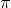Question

The Smith family has a circular shaped pool with a radius of 10 feet. What is the minimum amount of material needed to make a cover for the surface of the pool?

62.8 sq. feet

314 sq. feet

31.4 sq. feet

100 sq. feet

1.Philomena
314 sq. feet
Step-by-step explanation:
The surface area of the pool would be the area in math. The equation for solving the area of a circle goes as follows:
A=πr^2
π·10^2
314

2.A
Step-by-step explanation:
A circular shaped pool.
A =